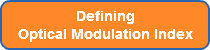### Importance & Definition of Optical Modulation Index (OMI)

What is Optical Modulation Index?  The primary factors in the performance of optical communications systems and specifically laser transmitters are Optical Power and Optical Modulation Index (OMI).  The OMI must be set properly to achieve optimum Carrier-to-Noise Ratio (CNR) while avoiding unacceptable large non-linear distortions and threshold clipping effects.  Static clipping behavior increases the lasers non-linear distortions and imposes an upper limit on its channel capacity.

The mathematical calculation of CNR for a multi-channel carrier system is a statistical calculation comprised of several variables: OMI (m), video noise BW (B), receiver photocurrent (I), receiver noise equivalent current (N), and laser Relative Intensity Noise (RIN):  CNR=(m*I)2/[2B(2qi+N+(RIN*I2)]

As you can see, OMI (m) is a key variable along with optical power in the CNR equation.  Therefore, in order to optimize the system, it is vital to get as much optical power from a laser as the result of setting OMI to the optimum level.

Since determining the optimal setting for a laser transmitter requires performing multiple calculations each time a variable changes, using a device like the FOS 1000A from M2 Optics that can quickly and automatically measure OMI can save significant time for an engineering team.

View Related Whitepaper: